Function Repository Resource:

# ParallelSurface

Compute the parallel surface of a surface

Contributed by: Wolfram Staff (original content by Alfred Gray)
 ResourceFunction["ParallelSurface"][s,r,{u,v}] computes the parallel surface at distance r from a parametric surface s in the variables u and v.

## Details and Options

A surface is parallel to another if, for each point of the first, there is a point on the second at distance r in the direction of the normal vector from that point. Each such pair will have parallel tangent planes.
A parallel surface is sometimes referred to as an offset surface.

## Examples

### Basic Examples (3)

Define a catenoid surface:

 In:=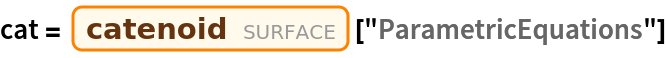Out=Compute a parallel surface to the catenoid:

 In:=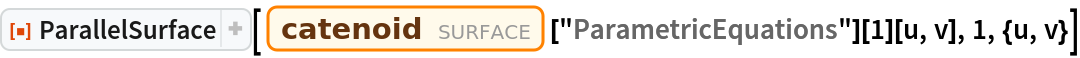Out=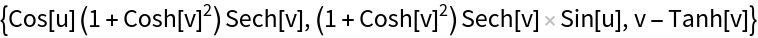Plot the surface and its parallels:

 In:=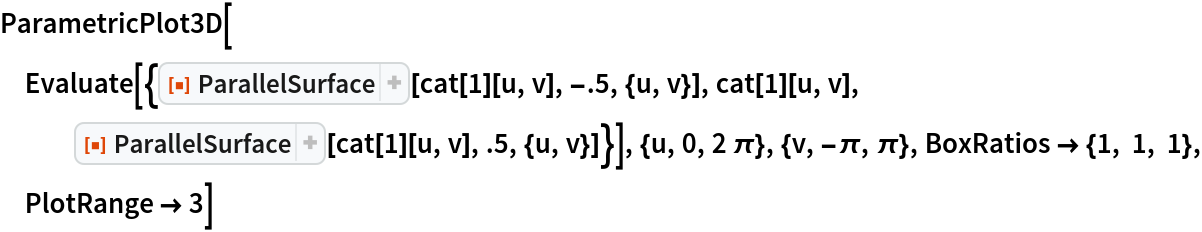Out=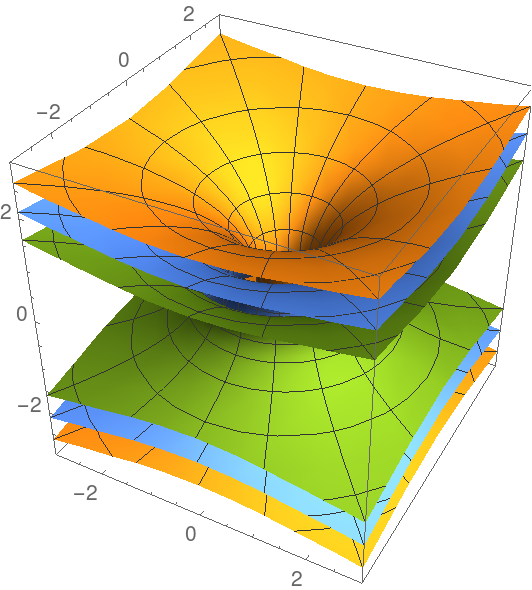### Scope (2)

Define an ellipsoid:

 In:=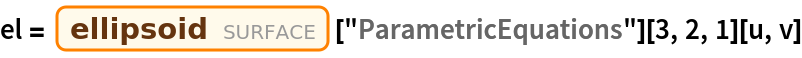Out=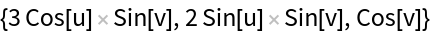Compute a parallel surface to the ellipsoid:

 In:=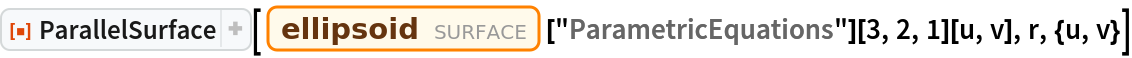Out=Plot the surface and its parallels with a cross section view:

 In:=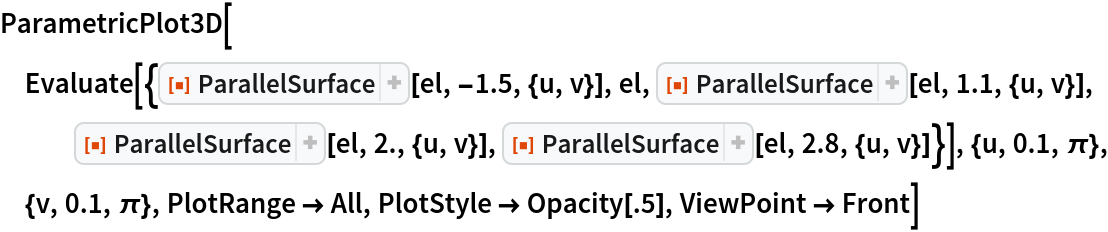Out=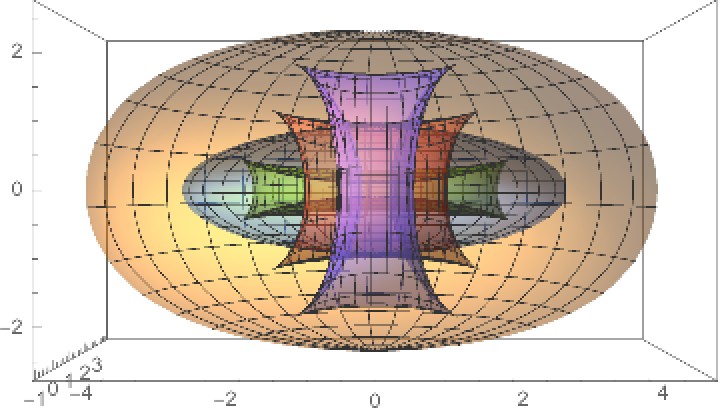Define a Möbius strip surface:

 In:=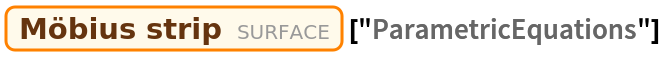Out=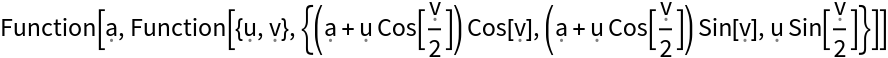Compute a parallel surface to the Möbius strip:

 In:=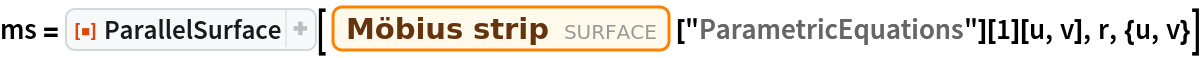Out=Plot the Möbius strip and a surface parallel to it, varying distance:

 In:=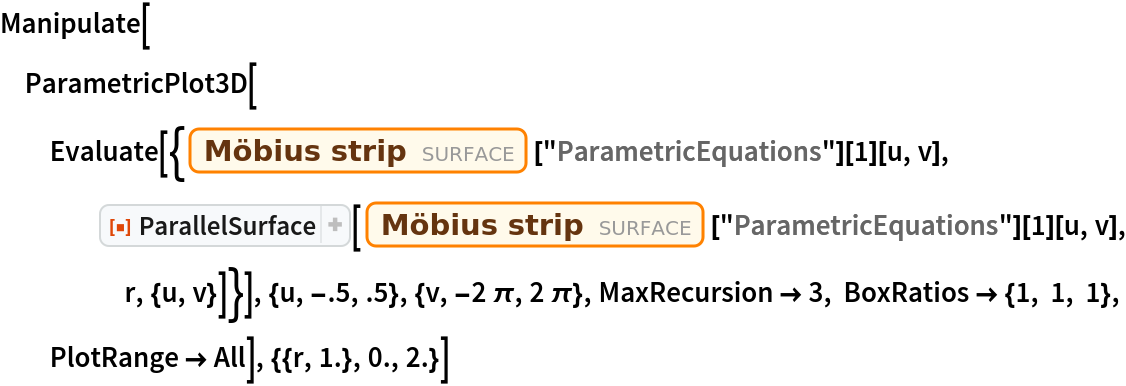Out=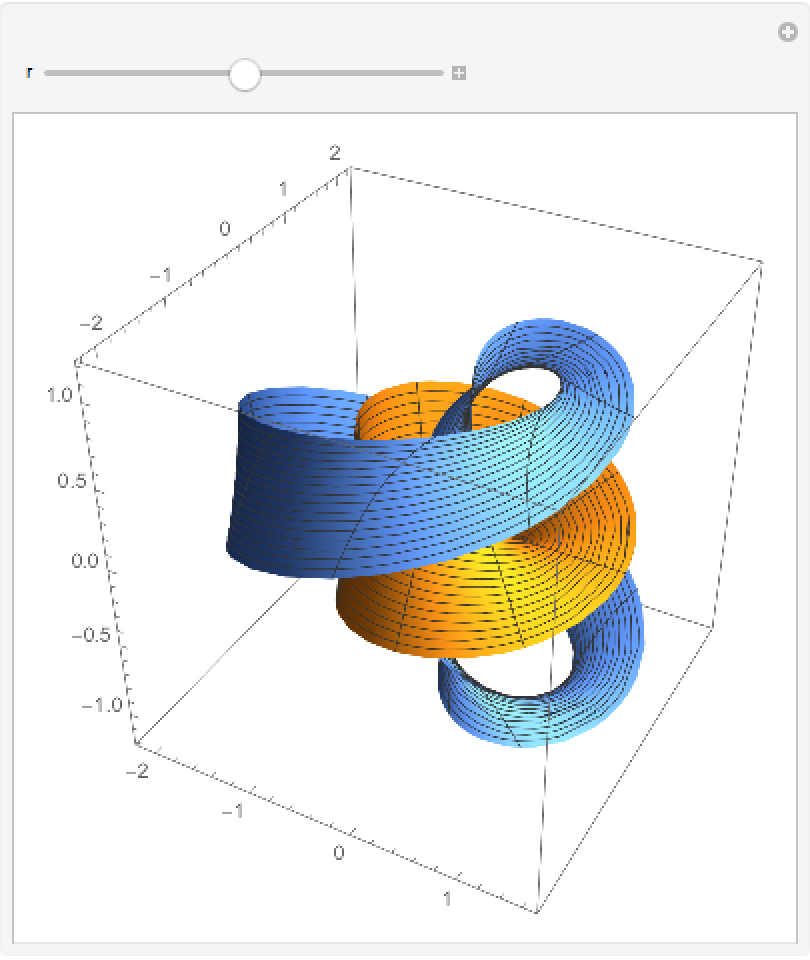Enrique Zeleny

## Version History

• 1.0.0 – 26 February 2020